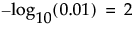The p-value for the significance test corresponding to the pair of Y and X variables. For details about Fit Y by X statistics, see Introduction to Fit Y by X of the Basic Analysis book.
The quantity -log10(p-value). This transformation adjusts p-values to provide an appropriate scale for graphing. A value that exceeds 2 is significant at the 0.01 level (because).
The False Discovery Rate p-value calculated using the Benjamini-Hochberg technique. This technique adjusts the p-values to control the false discovery rate for multiple tests. If there is no Group variable, the set of multiple tests includes all tests displayed in the table. If there is a Group variable, the set of multiple tests consists of all tests conducted for each level of the Group variable. For details about the FDR correction, see Benjamini and Hochberg, 1995. For details about the false discovery rate, see The False Discovery Rate.
The quantity -log10(FDR PValue). This is the best statistic for plotting and assessing significance. Note that small p-values result in high FDR LogWorth values. Cells corresponding to FDR LogWorth values greater than two (p-values less than 0.01) are colored with an intensity gradient.
 – When Y is categorical and X is continuous, the effect size is the square root of the average ChiSquare value for the whole model test.
 – When Y and X are both categorical, the effect size is the square root of the average Pearson ChiSquare.
The rank of the FDR LogWorth expressed as a fraction of the number of tests. If the number of tests is m, the largest FDR LogWorth value has Rank Fraction 1/m, and the smallest has Rank Fraction 1. Equivalently, the Rank Fraction ranks the p-values in increasing order, as a fraction of the number of tests. The Rank Fraction is used in plotting the PValues and FDR PValues in rank order of decreasing significance.

Help created on 9/19/2017